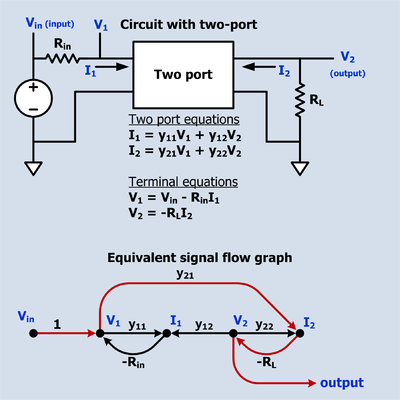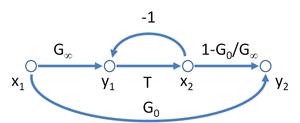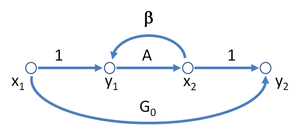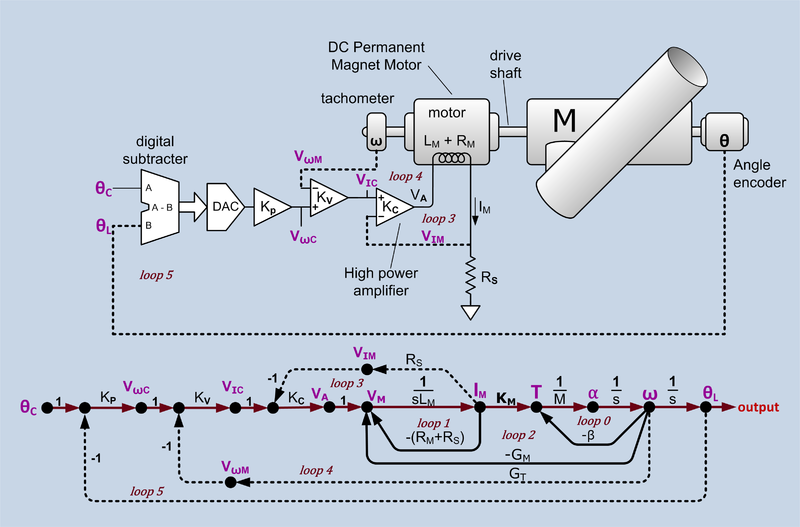# Signal-flow graph

Signal-flow graph

A signal-flow graph (SFG) is a special type of block diagram—and directed graph—consisting of nodes and branches. Its nodes are the variables of a set of linear algebraic relations. An SFG can only represent multiplications and additions. Multiplications are represented by the weights of the branches; additions are represented by multiple branches going into one node. A signal-flow graph has a one-to-one relationship with a system of linear equations. In addition to this, it can also be used to represent the signal flow in a physical system; i.e., it can represent relations of cause and effect.

## Example 1: Simple amplifierFigure 1: SFG of a simple amplifier

The amplification of a signal V1 by an amplifier with gain a12 is described mathematically by$V_2 = a_{12}V_1 \,.$

This relationship represented by the signal-flow graph of Figure 1. is that V2 is dependent on V1 but it implies no dependency of V1 on V2. See Kou page 57.

## Example 2: Two-port networkFigure 2: SFG describing two-port admittance matrix equations

In electrical circuits, two equations describing a two-port network in admittance matrix form,$\begin{bmatrix} I_1 \\ I_2 \end{bmatrix} = \begin{bmatrix} y_{11} & y_{12} \\ y_{21} & y_{22} \end{bmatrix} \begin{bmatrix} V_1 \\ V_2 \end{bmatrix}$

can be drawn as the SFG shown in Figure 2. This signal-flow graph describes causality properly if and only if V1 and V2 are designated as inputs. If any other designation of inputs is made then the SFG is either incomplete or invalid.

## Example 2a: Circuit containing a two-port networkSignal flow graph of a circuit containing a two port. The forward path from input to output is shown in a different color.

The figure to the right depicts a circuit that contains a two-port network. Vin is the input of the circuit and V2 is the output. The two-port equations impose a set of linear constraints between its port voltages and currents. The terminal equations impose other constraints. All these constraints are represented in the SFG (Signal Flow Graph) below the circuit. There is only one path from input to output which is shown in a different color and has a gain of -RLy21. There are also three loops: -Riny11, -RLy22, RinRLy12y21. Sometimes a loop indicates intentional feedback but it can also indicate a constraint on the relationship of two variables. For example, the equation that describes a resister says that the ratio of the voltage across the resister to the current through the resister is a constant which is called the resistance. This can be interpreted as the voltage is the input and the current is the output, or the current is the input and the voltage is the output, or merely that the voltage and current have a linear relationship. Virtually all passive two terminal devices in a circuit will show up in the SFG as a loop.

The SFG and the schematic depict the same circuit, but the schematic also suggests the circuit's purpose. Compared to the schematic, the SFG is awkward but it does have the advantage that the input to output gain can be written down by inspection using Mason's rule.

## Example 3 Asymptotic gain formulaFigure 3: A possible signal-flow graph for the asymptotic gain modelFigure 4: A different signal-flow graph for the asymptotic gain model
A possible SFG for the asymptotic gain model for a negative feedback amplifier is shown in Figure 3, and leads to the equation for the gain of this amplifier as$G = \frac {y_2}{x_1}$$= G_{\infty} \left( \frac{T}{T + 1} \right) + G_0 \left( \frac{1}{T + 1} \right) \ .$
The interpretation of the parameters is as follows: T = return ratio, G = direct amplifier gain, G0 = feedforward (indicating the possible bilateral nature of the feedback, possibly deliberate as in the case of feedforward compensation). Figure 3 has the interesting aspect that it resembles Figure 2 for the two-port network with the addition of the extra feedback relation x2 = T y1.
From this gain expression an interpretation of the parameters G0 and G is evident, namely:$G_{\infty} = \lim_{T \to \infty }G\ ; \ G_{0} = \lim_{T \to 0 }G \ .$
There are many possible SFG's associated with any particular gain relation. Figure 4 shows another SFG for the asymptotic gain model that can be easier to interpret in terms of a circuit. In this graph, parameter β is interpreted as a feedback factor and A as a "control parameter", possibly related to a dependent source in the circuit. Using this graph, the gain is$G = \frac {y_2}{x_1}$$= G_{0} + \frac {A} {1 - \beta A} \ .$
To connect to the asymptotic gain model, parameters A and β cannot be arbitrary circuit parameters, but must relate to the return ratio T by:$T = - \beta A \ ,$
and to the asymptotic gain as:$G_{\infty} = \lim_{T \to \infty }G = G_0 - \frac {1} {\beta} \ .$
Substituting these results into the gain expression,$G = G_{0} + \frac {1} {\beta} \frac {-T} {1 +T}$$= G_0 + (G_0 - G_{\infty} ) \frac {-T} {1 +T}$$= G_{\infty} \frac {T} {1 +T} + G_0 \frac {1}{1+T} \ ,$
which is the formula of the asymptotic gain model.

Signal flow graphs are used in many different subject areas besides control and network theory, for example, stochastic signal processing.

## Example 4: Position servo with multi-loop feedbackAngular position servo and signal flow graph. θC = desired angle command, θL = actual load angle, KP = position loop gain, VωC = velocity command, VωM = motor velocity sense voltage, KV = velocity loop gain, VIC = current command, VIM = current sense voltage, KC = current loop gain, VA = power amplifier output voltage, LM = motor inductance, VM = voltage across motor inductance, IM = motor current, RM = motor resistance, RS = current sense resistance, KM = motor torque constant (Nm/amp) , T = torque, M = momment of inertia of all rotating components α = angular acceleration, ω = angular velocity, β = mechanical damping, GM = motor back EMF constant, GT = tachometer conversion gain constant,. There is one forward path (shown in a different color) and six feedback loops. The drive shaft assumed to be stiff enough to not treat as a spring. Constants are shown in black and variables in purple.

This example is representative of a SFG (signal-flow graph) used to represent a servo control system and illustrates several features of SFGs. Some of the loops (loop 3, loop 4 and loop 5) are extrinsic intentionally designed feedback loops. These are shown with dotted lines. There are also intrinsic loops (loop 0, loop1, loop2) that are not intentional feedback loops, although they can be analyzed as though they were. These loops are shown with solid lines. Loop 3 and loop 4 are also known as minor loops because they are inside a larger loop.

• The forward path begins with$\theta_C \,$, the desired position command. This is multiplied by KP which could be a constant or a function of frequency. KP incorporates the conversion gain of the DAC and any filtering on the DAC output. The output of KP is the velocity command$V_{\omega C} \,$ which is multiplied by KV which can be a constant or a function of frequency. The output of KV is the current command, VIC which is multiplied by KC which can be a constant or a function of frequency. The output of KC the amplifier output voltage, VA. The current, IM, though the motor winding is the integral of the voltage applied to the inductance. The motor produces a torque, T, proportional to IM. Permanent magnet motors tend to have a linear current to torque function. The conversion constant of current to torque is KM. The torque, T, divided by the load moment of inertia, M, is the acceleration,$\alpha \,$, which is integrated to give the load velocity$\omega \,$ which is integrated to produce the load position,$\theta_L \,$.

• The forward path of loop 0 asserts that acceleration is proportional to torque and the velocity is the time integral of acceleration. The back ward path says that as the speed increases there is a friction or drag that counteracts the torque. Torque on the load decreases proportionately to the load velocity until the point is reached that all the torque is used to overcome friction and the acceleration drops to zero. Loop 0 is intrinsic.

• Loop1 represents the interaction of an inductor's current with its internal and external series resistance. The current through an inductance is the time integral of the voltage across the inductance. When a voltage is first applied, all of it appears across the inductor. This is shown by the forward path through$\frac {1} {s L_M} \,$. As the current increases, voltage is dropped across the inductor internal resistance RM and the external resistance RS. This reduces the voltage across the inductor and is represented by the feedback path -(RM + RS). The current continues to increase but at a steadily decreasing rate until the current reaches the point at which all the voltage is dropped across (RM + RS). Loop 1 is intrinsic.

• Loop2 expresses the effect of the motor back EMF. Whenever a permanent magnet motor rotates, it acts like a generator and produces a voltage in its windings. It does not matter whether the rotation is caused by a torque applied to the drive shaft or by current applied to the windings. This voltage is referred to as back EMF. The conversion gain of rotational velocity to back EMF is GM. The polarity of the back EMF is such that it diminishes the voltage across the winding inductance. Loop 2 is intrinsic.

• Loop 3 is extrinsic. The current in the motor winding passes through a sense resister. The voltage, VIM, developed across the sense resister is fed back to the negative terminal of the power amplifier KC. This feedback causes the voltage amplifier to act like a voltage controlled current source. Since the motor torque is proportional to motor current, the sub-system VIC to the output torque acts like a voltage controlled torque source. This sub-system may be referred to as the "current loop" or "torque loop". Loop 3 effectively diminishes the effects of loop 1 and loop 2.

• Loop 4 is extrinsic. A tachometer (actually a low power dc generator) produces an output voltage$V_ {\omega M} \,$ that is proportional to is angular velocity. This voltage is fed to the negative input of KV. This feedback causes the sub-system from$V_ {\omega C} \,$ to the load angular velocity to act like a voltage to velocity source. This sub-system may be referred to as the "velocity loop". Loop 4 effectively diminishes the effects of loop 0 and loop 3.

• Loop 5 is extrinsic. This is the overall position feedback loop. The feedback comes from an angle encoder that produces a digital output. The output position is subtracted from the desired position by digital hardware which drives a DAC which drives KP. In the SFG, the conversion gain of the DAC is incorporated into KP.

see Mason's rule for development of Mason's Gain Formula for this example.

Wikimedia Foundation. 2010.

### Look at other dictionaries:

• signal flow graph — signalinis grafas statusas T sritis automatika atitikmenys: angl. signal flow graph vok. Signalflußdiagramm, n; Wirkplan, m rus. сигнальный граф, m pranc. graphe de fluence des signaux, m …   Automatikos terminų žodynas

• Graph drawing — This article is about the general subject of graph drawing. For the annual research symposium, see International Symposium on Graph Drawing. Graphic representation of a minute fraction of the WWW, demonstrating hyperlinks. Graph drawing is an… …   Wikipedia

• Signal transduction — In biology, signal transduction refers to any process by which a cell converts one kind of signal or stimulus into another. Most processes of signal transduction involve ordered sequences of biochemical reactions inside the cell, which are… …   Wikipedia

• Bond graph — A bond graph is a graphical description of a physical dynamic system. It is an energy based graphical technique for building mathematical models of dynamic systems. A bond graph depicts the energy flow between components used to model a system.… …   Wikipedia

• Mason's rule — Mason s gain formula (MGF) is a method for finding the transfer function of a linear signal flow graph (SFG). The formula was derived by Samuel Jefferson Mason and is named after its discoverer. MGF is an alternate method to finding the… …   Wikipedia

• Minor loop feedback — is a classical method used to design stable robust linear Feedback control systems using feedback loops around sub systems within the over all feedback loop. The method is sometimes called minor loop synthesis in college text books, some …   Wikipedia

• Adel Sedra — is an Egyptian electrical engineer whose research specialization is microelectronics, with particular emphasis on applications in communication and instrumentation systems. He received his bachelor of science in electrical engineering from Cairo… …   Wikipedia

• Dataflow — This article is about software engineering. For the flow of data within a computer network see packet flow For the graphical representation of flow of data within an information system, see data flow diagram Dataflow is a term used in computing,… …   Wikipedia

• Symbolic Sound Corporation — was founded by Carla Scaletti and Kurt J. Hebel in 1989 as a spinoff of the CERL Sound Group at the Computer based Education Research Laboratory of the University of Illinois at Urbana Champaign. Originally named Kymatics , the company was… …   Wikipedia

• Asymptotic gain model — The asymptotic gain model [Middlebrook, RD: Design oriented analysis of feedback amplifiers ; Proc. of National Electronics Conference, Vol. XX, Oct. 1964, pp. 1 4] G {infin} T} . while in classical feedback theory, in terms of the open loop gain …   Wikipedia